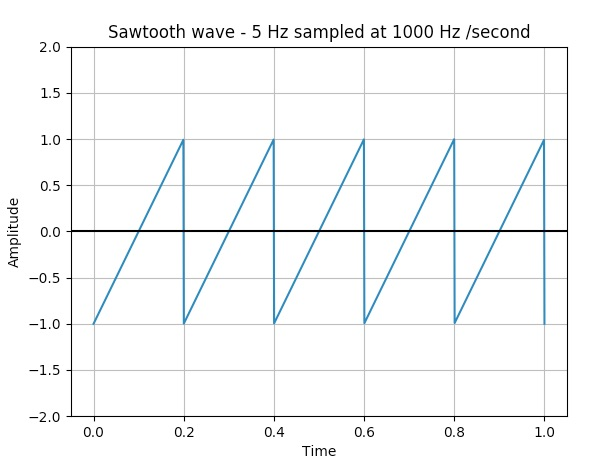# Plotting A Sawtooth Wave Using Scipy, Numpy And Matplotlib

## Overview:

• A sawtooth wave is a periodic waveform and it is non-sinusoidal.

• A sawtooth wave rises upwards and drops sharply.  A sawtooth wave can also go down and rise sharply which is called as  "reverse sawtooth wave" or "inverse sawtooth wave".

• Saw tooth waves have their applications in music synthesizers, in CRT based video displays and in Oscilloscopes.

• Sawtooth waves can be plotted using the python libraries scipy and matplolib.

• The function signal.sawtooth() returns a periodic sawtooth waveform or a triangular waveform.

## Example:

 # import the required python modules import numpy as np from scipy import signal import matplotlib.pyplot as plot   # Create 1000 linearly separated points with values between 0 to 1 timePoints = np.linspace(0, 1, 500)   # Sawtooth wave frequency 5Hz; sampling frequency 500 Hz plot.plot(timePoints, signal.sawtooth(2 * np.pi * 5 * timePoints))   # Give a title for the sawtooth wave plot plot.title('Sqaure wave - 5 Hz sampled at 1000 Hz /second')   # Give x axis label for the sawtooth wave plot plot.xlabel('Time')   # Give y axis label for the sawtooth wave plot plot.ylabel('Amplitude')   plot.grid(True, which='both')   # Provide x axis and line color plot.axhline(y=0, color='k')   # Set the max and min values for y axis plot.ylim(-2, 2)   # Display the sawtooth wave plotted plot.show()

## Output: